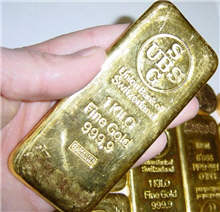Definition of

# MassA measure of how much matter is in an object.

This gold bar is quite small but has a mass of 1 kilogram (about 2.2 pounds), so it contains a lot of matter.

Mass is commonly measured by how much something weighs. But weight is caused by gravity, so your weight on the Moon is less than here on Earth, while the mass stays the same.

Mass is measured in grams, kilograms and, tonnes (Metric) or ounces and pounds (US units).
See: Weight